# Normal convergence

Convergence of a series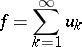(1)

formed by bounded mappings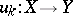from a setinto a normed space, such that the series with positive terms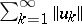formed by the norms of the mappings,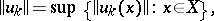converges.

Normal convergence of the series (1) implies absolute and uniform convergence of the series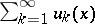consisting of elements of; the converse is not true. For example, if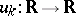is the real-valued function defined by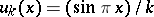for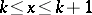and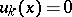for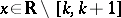, then the seriesconverges absolutely, whereasdiverges.

Suppose, in particular, that eachis a piecewise-continuous function on a non-compact interval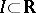and that (1) converges normally. Then one can integrate term-by-term on: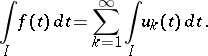Let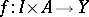, whereis an interval, have left and right limits at each point of. Then the improper integral(2)

is called normally convergent onif there exists a piecewise-continuous positive function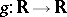such that: 1)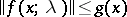for any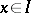and any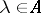; and 2) the integralconverges. Normal convergence of (2) implies its absolute and uniform convergence; the converse is not true.